# Normed Spaces and Tensor Products

Much of this chapter is preparation for what we will do later when we will prove that if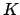is complete with respect to a valuation (and locally compact) and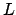is a finite extension of, then there is a unique valuation onthat extends the valuation on. Also, ifis a number field,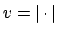is a valuation on,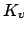is the completion ofwith respect to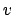, andis a finite extension of, we'll prove that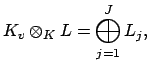where the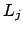are the completions ofwith respect to the equivalence classes of extensions ofto. In particular, ifis a number field defined by a root of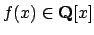, then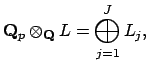where thecorrespond to the irreducible factors of the polynomial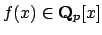(hence the extensions of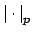correspond to irreducible factors of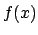over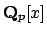).

In preparation for this clean view of the local nature of number fields, we will prove that the norms on a finite-dimensional vector space over a complete field are all equivalent. We will also explicitly construct tensor products of fields and deduce some of their properties.

Subsections
William Stein 2004-05-06